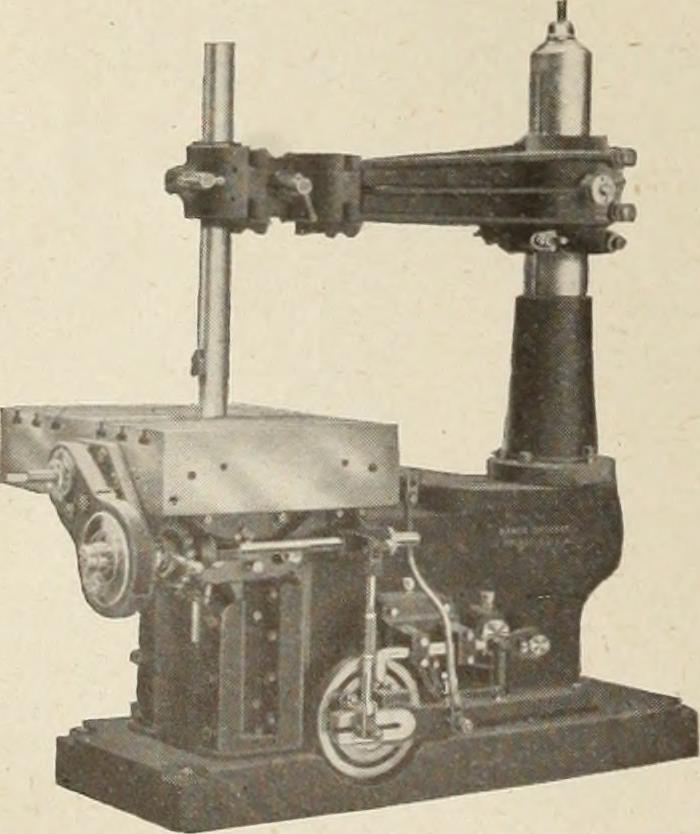#Are the Measures Failure Rate and Probability of Failure Different?

Failure rate and probability are similar. They are slightly different, too.

One of the problems with reliability engineering is so many terms and concepts are not commonly understood.

Reliability, for example, is commonly defined as dependable, trustworthy, as in you can count on him to bring the bagels. Whereas, reliability engineers define reliability as the probability of successful operation/function within in a specific environment over a defined duration.

The same for failure rate and probability of failure. We often have specific data-driven or business-related goals behind the terms. Others do not.
If we do not state over which time period either term applies, that is left to the imagination of the listener. Which is rarely good.

## Failure Rate Definition

There at least two failure rates that we may encounter: the instantaneous failure rate and the average failure rate. The trouble starts when you ask for and are asked about an item’s failure rate. Which failure rate are you both talking about?

The instantaneous failure rate is also known as the hazard rate h(t)

￼￼￼￼$\displaystyle h\left( t \right)=\frac{f\left( t \right)}{R\left( t \right)}$

Where f(t) is the probability density function and R(t) is the relaibilit function with is one minus the cumulative distribution function. The hazard rate, failure rate, or instantaneous failure rate is the failures per unit time when the time interval is very small at some point in time, t. Thus, if a unit is operating for a year, this calculation would provide the chance of failure in the next instant of time.

This is not useful for the calculation of the number of failures over that year, only the chance of a failure in the next moment.

The probability density function provides the fraction failure over an interval of time. As with a count of failures per month, a histogram of the count of failure per month would roughly describe a PDF, or f(t). The curve described for each point in time traces the value of the individual points in time instantaneous failure rate.

Sometimes, we are interested in the average failure rate, AFR. Where the AFR over a time interval, t1 to t2, is found by integrating the instantaneous failure rate over the interval and divide by t2 – t1. When we set t1 to 0, we have$\displaystyle AFR\left( T \right)=\frac{H\left( T \right)}{T}=\frac{-\ln R\left( T \right)}{T}$

Where H(T) is the integral of the hazard rate, h(t) from time zero to time T,
T is the time of interest which define a time period from zero to T,
And, R(T) is the reliability function or probability of successful operation from time zero to T.

A very common understanding of the rate of failure is the calculation of the count of failures over some time period divided by the number of hours of operation. This results in the fraction expected to fail on average per hour. I’m not sure which definition of failure rate above this fits, and yet find this is how most think of failure rate.

If we have 1,000 resistors that each operate for 1,000 hours, and then a failure occurs, we have 1 / (1,000 x 1,000 ) = 0.000001 failures per hour.

Let’s save the discussion about the many ways to report failure rates, AFR (two methods, at least), FIT, PPM/K, etc.

## Probability of Failure Definition

I thought the definition of failure rate would be straightforward until I went looking for a definition. It is with trepidation that I start this section on the probability of failure definition.

To my surprise it is actually rather simple, the common definition both in common use and mathematically are the same. There are two equivalent ways to phrase the definition:

1. The probability or chance that a unit drawn at random from the population will fail by time t.
2. The proportion or fraction of all units in the population that fail by time t.

We can talk about individual items or all of them concerning the probability of failure. If we have a 1 in 100 chance of failure over a year, then that means we have about a 1% chance that the unit we’re using will fail before the end of the year. Or it means if we have 100 units placed into operation, we would expect one of them to fail by the end of the year.

The probability of failure for a segment of time is defined by the cumulative distribution function or CDF.

## When to Use Failure Rate or Probability of Failure

This depends on the situation. Are you talking about the chance to failure in the next instant or the chance of failing over a time interval? Use failure rate for the former, and probability of failure for the latter.

In either case, be clear with your audience which definition (and assumptions) you are using. If you know of other failure rate or probability of failure definition, or if you know of a great way to keep all these definitions clearly sorted, please leave a comment below.

# Why Things Fail

Just a short note today about a great high level article in Wired magazine. Robert Capps did a nice summary and review of the significance of reliability engineering, product failure and what we can do about it.

And he doesn’t mention MTBF – which is appropriate.

http://www.wired.com/design/2012/10/ff-why-products-fail/all/

# International Day of Failure

In light of the International Day of Failure, Oct 13th, let’s consider failure from a reliability engineer’s point of view. We work to understand and avoid product failures. When a product fails to deliver the desired performance attribute it is tossed away, returned, replaced, repaired, or tolerated. This may occur before or after the value of the product has been achieved. Continue reading International Day of Failure